# A.1 Rotation transforms

Rotation matrices are used to describe the orientation of 3D coordinate frames in space, and to transform vectors between these coordinate frames.Figure A.1: Two coordinate frames A and B rotated with respect to each other.

Consider two 3D coordinate frames A and B that are rotated with respect to each other (Figure A.1). The orientation of B with respect to A can be described by a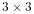rotation matrix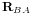, whose columns are the unit vectors giving the directions of the rotated axes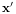,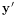, and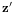of B with respect to A.is an orthogonal matrix, meaning that its columns are both perpendicular and mutually orthogonal, so that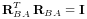(A.1)

where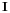is theidentity matrix. The inverse ofis hence equal to its transpose: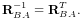(A.2)

Becauseis orthogonal,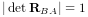, and because it is a rotation,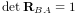(the other case, where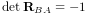, is not a rotation but a reflection). The 6 orthogonality constraints associated with a rotation matrix mean that in spite of having 9 numbers, the matrix only has 3 degrees of freedom.

Now, assume we have a 3D vector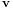, and consider its coordinates with respect to both frames A and B. Where necessary, we use a preceding superscript to indicate the coordinate frame with respect to which a quantity is described, so that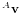and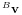and denotewith respect to frames A and B, respectively. Given the definition of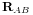given above, it is fairly straightforward to show that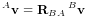(A.3)

and, given (A.2), that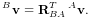(A.4)

Hence in addition to describing the orientation of B with respect to A,is also a transformation matrix that maps vectors in B to vectors in A.

It is straightforward to show that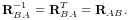(A.5)Figure A.2: Schematic illustration of three coordinate frames A, B, and C and the rotational transforms relating them.

A simple rotation by an angle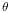about one of the basic coordinate axes is known as a basic rotation. The three basic rotations about x, y, and z are: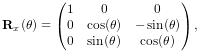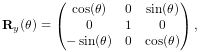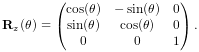Next, we consider transform composition. Suppose we have three coordinate frames, A, B, and C, whose orientation are related to each other by,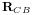, and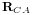(Figure A.6). If we knowand, then we can determinefrom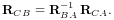(A.6)

This can be understood in terms of vector transforms.transforms a vector from C to B, which is equivalent to first transforming from C to A,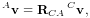(A.7)

and then transforming from A to B: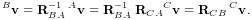(A.8)

Note also from (A.5) thatcan be expressed as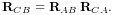(A.9)

In addition to specifying rotation matrix components explicitly, there are numerous other ways to describe a rotation. Three of the most common are:

Roll-pitch-yaw angles

There are 6 variations of roll-pitch-yaw angles. The one used in ArtiSynth corresponds to older robotics texts (e.g., Paul, Spong) and consists of a roll rotation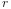about the z axis, followed by a pitch rotation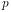about the new y axis, followed by a yaw rotation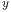about the new x axis. The net rotation can be expressed by the following product of basic rotations: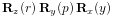.

Axis-angle

An axis angle rotation parameterizes a rotation as a rotation by an angleabout a specific axis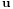. Any rotation can be represented in such a way as a consequence of Euler’s rotation theorem.

Euler angles

There are 6 variations of Euler angles. The one used in ArtiSynth consists of a rotation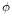about the z axis, followed by a rotationabout the new y axis, followed by a rotation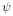about the new z axis. The net rotation can be expressed by the following product of basic rotations: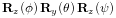.